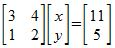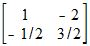# Multiplicative Inverses of Matrices and Matrix Equations

An error occurred trying to load this video.

Try refreshing the page, or contact customer support.

Coming up next: How to Take a Determinant of a Matrix

### You're on a roll. Keep up the good work!

Replay
Your next lesson will play in 10 seconds
• 0:02 The Matrix…
• 1:25 A Matrix Equation
• 2:39 Using the…
• 3:37 Lesson Summary
Save Save

Want to watch this again later?

Timeline
Autoplay
Autoplay
Speed Speed Audio mode

#### Recommended Lessons and Courses for You

Lesson Transcript
Instructor: Yuanxin (Amy) Yang Alcocer

Amy has a master's degree in secondary education and has taught math at a public charter high school.

Watch this video lesson to learn about another method you can use to solve a matrix problem if you are given the inverse of the matrix. You will also learn the identifying mark of the multiplicative inverse of a matrix.

## The Matrix Multiplicative Inverse

The multiplicative inverse of a matrix is the matrix that gives you the identity matrix when multiplied by the original matrix. In math symbol speak, we have A * A sup -1 = I. This tells you that when you multiply a matrix A with its multiplicative inverse, you will get the identity matrix.

Yes, we write the inverse with a superscript of -1. When we deal with regular numbers, our multiplicative inverse is the number we multiply by to get 1. So, for the number 2, it is 1/2. For the number -3, the multiplicative inverse is -1/3. For our normal numbers, the multiplicative inverse is simply 1 divided by our number.

Unfortunately, not all matrices will have an inverse, nor is finding the multiplicative inverse that simple. In order to find the multiplicative inverse, we have to find the matrix for which, when we multiply it with our matrix, we get the identity matrix. Our matrices must also be square, having the same number of rows and columns.

We will leave the discussion on how to find the inverse of a matrix to another lesson. For this lesson, we will talk about its benefits. You see, it is useful to learn about the multiplicative inverse of a matrix because if we know it, then we can use it to help us solve equations with matrices in them.

## A Matrix Equation

For example, we can use it to solve a problem like this:This matrix equation is in the form of Ax = b, where A is your coefficient matrix, x is your variable matrix, and b is your answer matrix. While we can use other methods to solve such a problem, if we know the multiplicative inverse of our coefficient matrix, then we can easily solve the problem by simply multiplying both sides by the inverse.

So, if we knew A sup -1, our answer would be x = A sup -1 * b. Yes, our answer would be our answer matrix, b, multiplied by the multiplicative inverse of our coefficient matrix. Let's look at how this works. For our coefficient matrix, we have this matrix as the multiplicative inverse matrix:We can check whether this inverse is real or not by multiplying it with our coefficient matrix to see if we get the identity matrix. Multiplying the two matrices, we see that we do get the identity matrix:We know for sure now that this inverse is the real inverse, and it works for us.

To unlock this lesson you must be a Study.com Member.

### Register to view this lesson

Are you a student or a teacher?

#### See for yourself why 30 million people use Study.com

##### Become a Study.com member and start learning now.
Back
What teachers are saying about Study.com

### Earning College Credit

Did you know… We have over 200 college courses that prepare you to earn credit by exam that is accepted by over 1,500 colleges and universities. You can test out of the first two years of college and save thousands off your degree. Anyone can earn credit-by-exam regardless of age or education level.